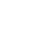Live chatIt is important for fourth graders to understand the concept of equivalence in order for them to learn how to deal with fractions containing unlike denominators. Equivalent fractions have the same value since if you multiply the numerator and denominator by a common value, the resultant fraction is the same as the original function. For fourth graders to understand this concept, they need to possess certain perquisite skills. These skills include differentiating between numerator and denominator, multiplying accurately and dividing accurately. This essay focuses on three perquisite skills that students need to possess in order to deal with unlike denominators. It also explains how concept of finding equivalence can be introduced using manipulatives. Furthermore, it describes the steps of finding equivalent functions in addition to transiting from complex manipulatives to representative paper and pencil problems. Lastly, it gives six equivalent equations I would use to test the transition.There are several prerequisite skills for working with fractions with unlike denominators. Students learning these fractions should have the ability of dividing numbers accurately. This is because this skill will enable the students to divide the numerator and denominator with the same number and this will enable them to express the equivalent function in the smallest terms possible. This will help the students to solve the problem with ease. The students should also have the skills of counting all the fractional parts. This will help the students to order the fractions with unlike denominators appropriately. Furthermore, it will help the student to develop improper fractions and mixed numbers that he may use to carry several fractional operations. The students should also possess the skill of multiplying different numbers. This skill will enable the students to multiply unlike denominators with ease. Furthermore, it will help in ensuring the students avoid any errors in that may result from improper multiplication.

Limited time Offer

0
0
days
:
0
0
hours
:
0
0
minutes
:
0
0
seconds
Get 19% OFF

The concept of finding equivalent functions could be introduced using manipulatives in the following ways. Manipulatives are physical objects used by teachers teaching mathematics to engage students using hands on learning. Examples of manipulatives include counters, place-value mats, fractional strips and money. In teaching the concept of equivalent functions, I will mainly concentrate on paper folding as a manipulative, which will help in fostering the understanding of this concept. I will ask the students to fold a piece of paper in half in one direction and half again in the other direction. I will then ask the students to unfold the paper and tell me the number of parts that they can observe. The number of parts will be four. After this, I will ask the students to color one of the parts and call it a fourth. I will then instruct the students to fold the paper again into half and unfold it to observe the number of parts that they can see. The number of parts will be eight and I will tell them to name these parts eighths. I will then ask the students to count the number of colored parts. These parts will be two. Introducing the concept of equivalent fractions, I will ask the students to tell me how many eighths are there in one fourth. The students will be able to know this answer since they will observe it using the folded sheet.

Stay ConnectedStudents should follow several steps in order to find equivalent fractions. In order for students to find equivalents with small terms, the students should divide the numerator and the denominator by a common factor.  This operation retains the equivalency of the fractions. However, the students should ensure that both the numerator and the denominator are divisible by this factor. This is to ensure easy simplification of the function since a fraction cannot be simplified if the numerator or denominator is not divisible by the common factor. Furthermore, each student should use the greatest common factor of both the numerator and denominator in order to ensure that they simply the fraction to its lowest equivalent term. To find equivalents of a fraction with larger terms, the students should multiply the numerator and denominator with any number if the number is unspecified. If the denominator is specified, the student should try finding which number should be multiplied to get the specified denominator. However, the students should consider finding out whether the dominator can be wholly multiplied by another number in order to get a specified denominator without leaving any fractions.

Benefit from Our Service: Save 25% Along with the first order offer - 15% discount, you save extra 10% since we provide 300 words/page instead of 275 words/page

I will help students to transit from concrete manipulatives to more representative paper and pencil problems by following this procedure. I will give each student different functional circle. These circles will be representing equivalent halves, fourths, sixths and eighths. I will then give each student a paper and pencil and instruct them to write their observations after completing the exercise that I will give them. In this exercise, I will instruct each student to take one-half piece of the fraction circle and try fitting as many sixths on it until it is full. I will ask the students to write the number of sixths that filled the one-half circle.

To conclude, students need the prerequisite skills of dividing numbers accurately, counting fractional parts and multiplying numbers accurately. The concept of equivalence could be introduced using the manipulative of paper folding. Students should follow a certain determined procedure in order to find equivalent fractions. In addition to this, I will use functional circles, a paper and a pencil in order to help the students to transit from concrete manipulatives to more representative paper and pencil problems.

0

Preparing Orders

0

Active Writers

0%

Positive Feedback

0

Support Agents

Now Accepting Apple Pay!
Limited offerget 15% off your 1st order with code first15